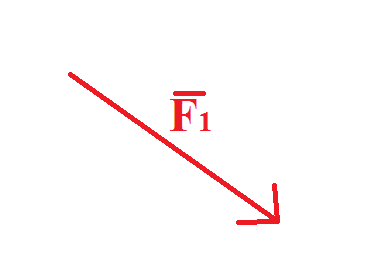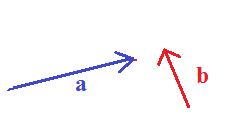# Vector Subtraction (Geometric): Formula & Examples - Quiz & Worksheet

Instructions:

question 1 of 3

### If the following image is the vector F1, what would negative F1 look like?Create Your Account To Take This Quiz

As a member, you'll also get unlimited access to over 84,000 lessons in math, English, science, history, and more. Plus, get practice tests, quizzes, and personalized coaching to help you succeed.

Try it risk-free for 30 days. Cancel anytime

### 1. Which of the following shows the correct result vector of vector a minus vector b?### 2. Which of these equations is a case where you would NOT ever need to subtract two vectors?

Create your account to access this entire worksheet
Quizzes, practice exams & worksheets
Certificate of Completion
Create an account to get started

This worksheet and quiz will gauge your understanding of vector subtraction. Answer questions on negative vectors and interpret vector a minus vector b.

## Quiz & Worksheet Goals

• Understand a case where you would not need to subtract two vectors
• Find a vector in a given problem
• Recognize when you would need to subtract two vectors

## Skills Practiced

Practice the following study skills:

• Reading comprehension - ensure that you draw the most important information from the related lesson on vector subtraction
• Critical thinking - apply relevant concepts to examine information about when you would need to subtract vectors in a different light
• Information recall - access the knowledge you've gained regarding negative vectors

Read this lesson on subtracting vectors for more information. The lesson is entitled Vector Subtraction (Geometric): Formula & Examples and it adheres to the following study points:

• Understand why subtracting vectors is necessary
• Define scale drawing
• Analyze real life examples of subtracting vectors
Final ExamUExcel Physics: Study Guide & Test Prep
Status: Not Started
Chapter ExamVectors
Status: Not Started

Support